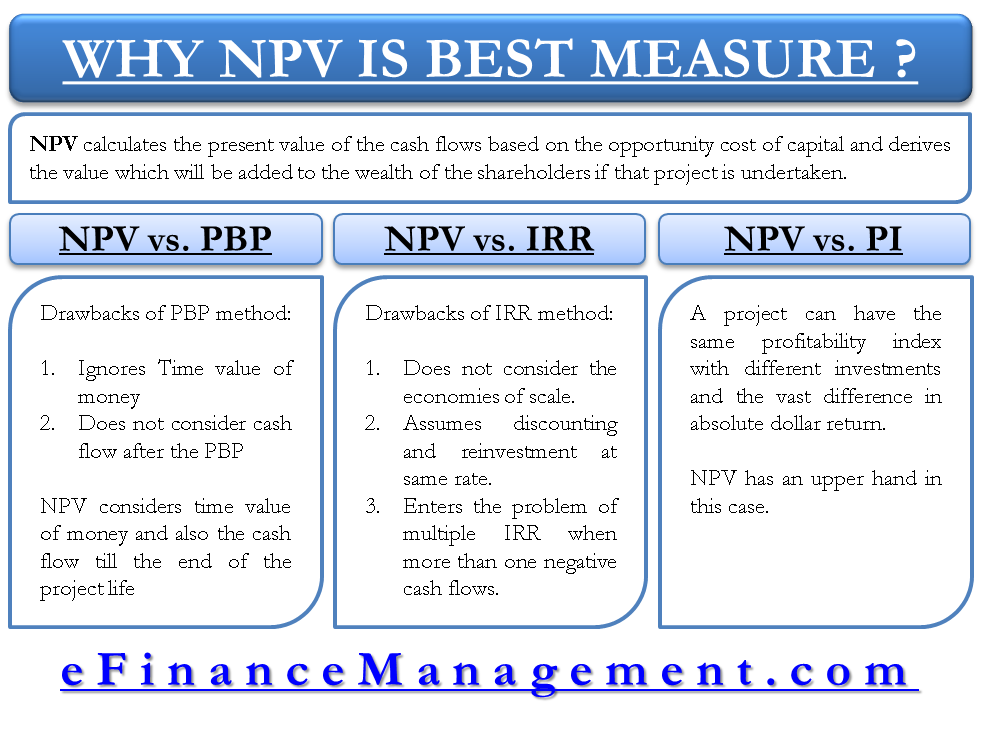# Why Net Present Value is the Best Measure for Investment Appraisal?

“Why net present value (NPV) is the best measure for investment appraisal?” This question is as good as another question – “How NPV is better than other methods of investment appraisal? There are many methods for investment appraisal such as accounting the (book) rate of return, payback period (PBP), internal rate of return (IRR), and Profitability Index (PI).

Before comparing NPV, let’s recapitulate the concept again. Net present value method calculates the present value of the cash flows based on the opportunity cost of capital and derives the value which will be added to the wealth of the shareholders if that project is undertaken.

Let us discuss each of these methods in comparison with net present value (NPV) to reach the conclusion.

## Net Present Value vs. Payback Period (NPV vs. PBP)

Payback period calculates a period within which the initial investment of the project is recovered.

The criterion for acceptance or rejection is just a benchmark decided by the firm say 3 Years. If the PBP is less than or equal to 3 Years, the firm will accept the project and else will reject it. Thereare two major drawbacks with this technique –

1. It does not consider the cash flows after the PBP.
2. Ignores time value of money.

The second drawback is still covered a bit by an extended version of PBP which is commonly called as Discounted Payback Period. The only difference it makes is the cash flows used are discounted cash flows but it also does not consider the cash flows after PBP.

Net present value considers the time value of money and also takes care of all the cash flows till the end of life of the project.

## Net Present Value vs. Internal Rate of Return (NPV vs. IRR)

The internal rate of return (IRR) calculates a rate of return which is offered by the project irrespective of the required rate of return and any other thing. It also has certain disadvantages discussed below:

1. IRR does not understand economies of scale and ignores the dollar value of the project. It cannot differentiate between two projects with same IRR but vast difference between dollar returns. On the other hand, NPV talks in absolute terms and therefore this point is not missed.
2. IRR assumes discounting and reinvestment of cash flows at the same rate. If the IRR of a very good project is say 35%, it is practically not possible to invest money at this rate in the market. Whereas, NPV assumes a rate of borrowing as well as lending near to the market rates and not absolutely impractical.
3. IRR enters the problem of multiple IRR when we have more than one negative net cash flow and the equation is then satisfied with two values, therefore, have multiple IRRs. Such a problem does not exist with NPV.## Net Present Value vs. Profitability Index (NPV vs. PI)

Profitability index is a ratio between the discounted cash inflow to the initial cash outflow. It presents a value which says how many times of the investment is the returns in the form of discounted cash flows.

The disadvantage associated with this method again is its relativity. A project can have the same profitability index with different investments and the vast difference in absolute dollar return. NPV has an upper hand in this case.

## Why is NPV the Best Method?

We have noted that almost all the difficulties are survived by net present value and that is why it is considered to be the best way to analyze, evaluate, and select big investment projects. At the same time, the estimation of cash flows requires carefulness because if the cash flow estimation is wrong, NPV is bound to be misleading.

A small problem with NPV is that it also considers the same discounting rate for both cash inflow and outflows. We know that there are differences between borrowing and lending rates. Modified internal rate of return is another method which is little more complex but improved which takes care of the difference between borrowing and lending rates also as it discounts cash inflows at lending rates and cash outflow at borrowing rates.

Last updated on : March 23rd, 2019
1.bello
2.Dr Kannan Arjunan